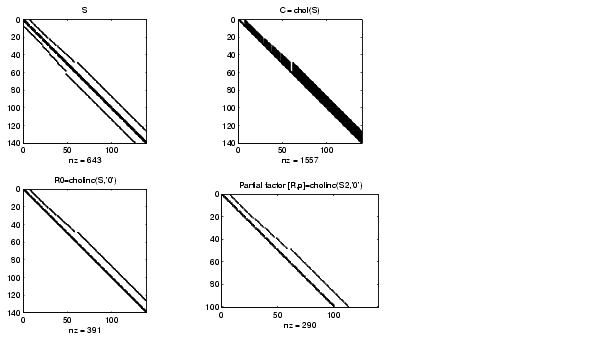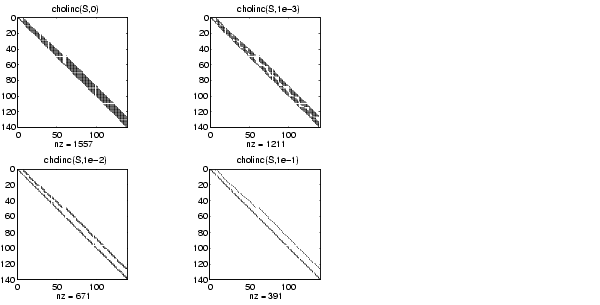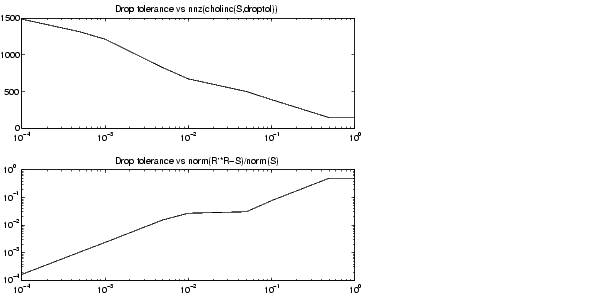MATLAB Function Referencecholinc

Sparse incomplete Cholesky and Cholesky-Infinity factorizations

Syntax

• ```R = cholinc(X,droptol)
R = cholinc(X,options)
R = cholinc(X,'0')
[R,p] = cholinc(X,'0')
R = cholinc(X,'inf')
```

Description

`cholinc` produces two different kinds of incomplete Cholesky factorizations: the drop tolerance and the 0 level of fill-in factorizations. These factors may be useful as preconditioners for a symmetric positive definite system of linear equations being solved by an iterative method such as `pcg` (Preconditioned Conjugate Gradients). `cholinc` works only for sparse matrices.

```R = cholinc(X,droptol) ``` performs the incomplete Cholesky factorization of `X`, with drop tolerance `droptol`.

```R = cholinc(X,options) ``` allows additional options to the incomplete Cholesky factorization. `options` is a structure with up to three fields:

 `droptol` Drop tolerance of the incomplete factorization `michol` Modified incomplete Cholesky `rdiag` Replace zeros on the diagonal of `R`

Only the fields of interest need to be set.

`droptol` is a non-negative scalar used as the drop tolerance for the incomplete Cholesky factorization. This factorization is computed by performing the incomplete LU factorization with the pivot threshold option set to `0` (which forces diagonal pivoting) and then scaling the rows of the incomplete upper triangular factor, `U`, by the square root of the diagonal entries in that column. Since the nonzero entries `U(i,j)` are bounded below by `droptol*norm(X(:,j))` (see `luinc`), the nonzero entries `R(i,j)` are bounded below by the local drop tolerance `droptol*norm(X(:,j))/R(i,i)`.

Setting `droptol = 0` produces the complete Cholesky factorization, which is the default.

`michol` stands for modified incomplete Cholesky factorization. Its value is either `0` (unmodified, the default) or `1` (modified). This performs the modified incomplete LU factorization of `X` and scales the returned upper triangular factor as described above.

`rdiag` is either `0` or `1`. If it is `1`, any zero diagonal entries of the upper triangular factor R are replaced by the square root of the local drop tolerance in an attempt to avoid a singular factor. The default is `0`.

```R = cholinc(X,'0') ``` produces the incomplete Cholesky factor of a real sparse matrix that is symmetric and positive definite using no fill-in. The upper triangular `R` has the same sparsity pattern as `triu(X`), although `R` may be zero in some positions where `X` is nonzero due to cancellation. The lower triangle of `X` is assumed to be the transpose of the upper. Note that the positive definiteness of `X` does not guarantee the existence of a factor with the required sparsity. An error message results if the factorization is not possible. If the factorization is successful, `R'*R` agrees with `X` over its sparsity pattern.

```[R,p] = cholinc(X,'0') ``` with two output arguments, never produces an error message. If `R` exists, `p` is `0`. If `R` does not exist, then `p` is a positive integer and `R` is an upper triangular matrix of size `q`-by-`n` where `q = p-1`. In this latter case, the sparsity pattern of `R` is that of the `q`-by-`n` upper triangle of `X`.` R'*R` agrees with `X` over the sparsity pattern of its first `q` rows and first `q` columns.

```R = cholinc(X,'inf') ``` produces the Cholesky-Infinity factorization. This factorization is based on the Cholesky factorization, and additionally handles real positive semi-definite matrices. It may be useful for finding a solution to systems which arise in interior-point methods. When a zero pivot is encountered in the ordinary Cholesky factorization, the diagonal of the Cholesky-Infinity factor is set to `Inf` and the rest of that row is set to `0`. This forces a `0` in the corresponding entry of the solution vector in the associated system of linear equations. In practice, `X` is assumed to be positive semi-definite so even negative pivots are replaced with a value of `Inf`.

Remarks

The incomplete factorizations may be useful as preconditioners for solving large sparse systems of linear equations. A single `0` on the diagonal of the upper triangular factor makes it singular. The incomplete factorization with a drop tolerance prints a warning message if the upper triangular factor has zeros on the diagonal. Similarly, using the `rdiag` option to replace a zero diagonal only gets rid of the symptoms of the problem, but it does not solve it. The preconditioner may not be singular, but it probably is not useful, and a warning message is printed.

The Cholesky-Infinity factorization is meant to be used within interior-point methods. Otherwise, its use is not recommended.

Examples

Example 1.

Start with a symmetric positive definite matrix, `S`.

• ```S = delsq(numgrid('C',15));
```

S is the two-dimensional, five-point discrete negative Lapacian on the grid generated by numgrid('C',15).

Compute the Cholesky factorization and the incomplete Cholesky factorization of level 0 to compare the fill-in. Make `S` singular by zeroing out a diagonal entry and compute the (partial) incomplete Cholesky factorization of level `0`.

• ```C = chol(S);
R0 = cholinc(S,'0');
S2 = S; S2(101,101) = 0;
[R,p] = cholinc(S2,'0');
```

Fill-in occurs within the bands of `S` in the complete Cholesky factor, but none in the incomplete Cholesky factor. The incomplete factorization of the singular `S2` stopped at row `p = 101` resulting in a 100-by-139 partial factor.

• ```D1 = (R0'*R0).*spones(S)-S;
D2 = (R'*R).*spones(S2)-S2;
```

`D1` has elements of the order of `eps`, showing that `R0'*R0` agrees with `S` over its sparsity pattern. `D2` has elements of the order of `eps` over its first 100 rows and first 100 columns, `D2(1:100,:)` and `D2(:,1:100)`.Example 2.

The first subplot below shows that `cholinc(S,0)`, the incomplete Cholesky factor with a drop tolerance of `0`, is the same as the Cholesky factor of `S`. Increasing the drop tolerance increases the sparsity of the incomplete factors, as seen below.Unfortunately, the sparser factors are poor approximations, as is seen by the plot of drop tolerance versus `norm(R'*R-S,1)/norm(S,1)` in the next figure.Example 3.

The Hilbert matrices have (i,j) entries 1/(i+j-1) and are theoretically positive definite:

• ```H3 = hilb(3)
H3 =
1.0000    0.5000    0.3333
0.5000    0.3333    0.2500
0.3333    0.2500    0.2000
R3 = chol(H3)
R3 =
1.0000    0.5000    0.3333
0    0.2887    0.2887
0         0    0.0745
```

In practice, the Cholesky factorization breaks down for larger matrices:

• ```H20 = sparse(hilb(20));
[R,p] = chol(H20);
p =
14
```

For `hilb(20)`, the Cholesky factorization failed in the computation of row 14 because of a numerically zero pivot. You can use the Cholesky-Infinity factorization to avoid this error. When a zero pivot is encountered, `cholinc` places an `Inf` on the main diagonal, zeros out the rest of the row, and continues with the computation:

• ```Rinf = cholinc(H20,'inf');
```

In this case, all subsequent pivots are also too small, so the remainder of the upper triangular factor is:

• ```full(Rinf(14:end,14:end))
ans =
Inf     0     0     0     0     0     0
0   Inf     0     0     0     0     0
0     0   Inf     0     0     0     0
0     0     0   Inf     0     0     0
0     0     0     0   Inf     0     0
0     0     0     0     0   Inf     0
0     0     0     0     0     0   Inf
```

Limitations

`cholinc` works on square sparse matrices only. For `cholinc(X,'0')` and `cholinc(X,'inf')`, `X` must be real.

Algorithm

`R = cholinc(X,droptol)` is obtained from `[L,U] = luinc(X,options)`, where `options.droptol = droptol` and `options.thresh = 0`. The rows of the uppertriangular `U` are scaled by the square root of the diagonal in that row, and this scaled factor becomes `R`.

`R = cholinc(X,options)` is produced in a similar manner, except the `rdiag` option translates into the `udiag` option and the `milu` option takes the value of the `michol` option.

`R = cholinc(X,'0')` is based on the "KJI" variant of the Cholesky factorization. Updates are made only to positions which are nonzero in the upper triangle of `X`.

`R = cholinc(X,'inf')` is based on the algorithm in Zhang .

See Also

`chol`, `luinc`, `pcg`

References

  Saad, Yousef, Iterative Methods for Sparse Linear Systems, PWS Publishing Company, 1996. Chapter 10, "Preconditioning Techniques."

  Zhang, Yin, Solving Large-Scale Linear Programs by Interior-Point Methods Under the MATLAB Environment, Department of Mathematics and Statistics, University of Maryland Baltimore County, Technical Report TR96-01

© 1994-2005 The MathWorks, Inc.